In an abstract algebra course, the Chinese Remainder Theorem says that if two positive integers,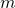and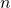, are relatively prime, then the ring of integers mod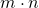is isomorphic to the direct product the rings of integers modand.
In number theory, the same fact is framed differently, that the system of congruences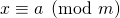and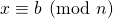always has a unique solution mod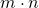as long asandare relatively prime.
My dissertation research involved approximation and interpolation of functions, and when you generalize the Chinese Remainder Theorem to Euclidean Domains, one immediate implication is that given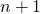points on the plane (pick any field) with distinct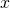-values, there is always a unique polynomial of degreeor less that passes through the points.